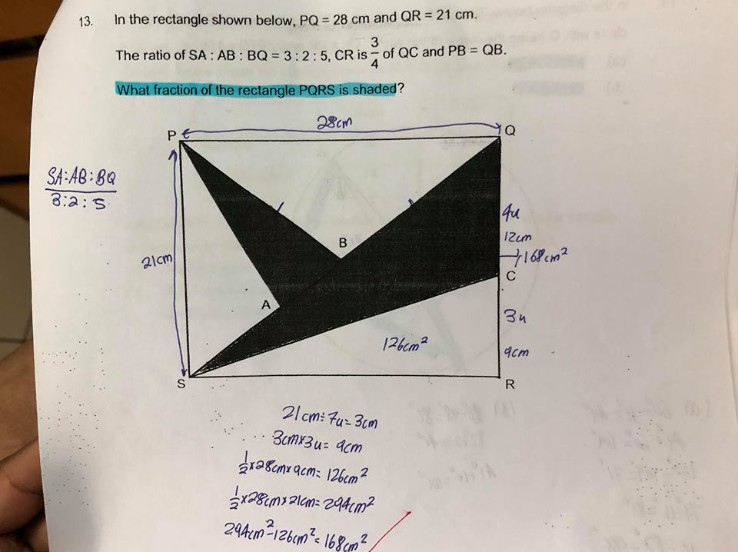# QuestionArea of rectangle = 21 × 28 = 588

Since B is the midpoint of the diagonal QS, the perpendicular distance of B from PS must be half of PQ. So area of triangle PAS = 0.5 × 21 × 3/5 × 14 = 88.2

Area of triangle PQB = 0.5 × 28 × 10.5  = 147

Area of triangle SRC = 126

Shaded area = 588 – 88.2 – 147 – 126 = 226.8

Fraction that is shaded = 226.8/588 = 27/70

PAS = 0.5 × 21 × 3/5 × 14 = 88.2

how u get 3/5?

Ratio is 3:2.

Point B is the midpoint..

Half 0f 28 is 14.

Perpendicular height is 3/5 of 14 cm.

Or 3/10 of 28 cm.

understood thanks

As a courtesy, please accept the answer if you agree with it.

Thank you.

0 Replies 0 Likes

Hello, my nephew is searching for this answer o but he can’t find it anywhere. He asked me to help him out but I am busy searching for a business plan for bank loan online. But I am glad I have found your post and I will happily share this post with my nephew.

0 Replies 0 Likes

Another way of doing this is by calculating the fraction.

Area of rectangle = L × B

Area of triangle SRC = 1/2 × L × 3/7 B = 3/14 L×B

Area of triangle PQB = 1/2 × L × 1/2 B = 1/4 L × B

Area of triangle PSA = 1/2 × B × 3/10 L = 3/20 L × B

Area of unshaded part = (3/14 + 1/4 + 3/20) L × B  = 43/70 L × B

Area of shaded part = (1 – 43/70) L × B = 27/70 L × B

Fraction that is shaded is 27/70.